## Tuesday, February 19, 2013

### A Word Problem

Mr. Subjunctive has 38 seeds to start in vermiculite.1 The container he wants to start them in is an undivided rectangle measuring 12 inches by 8 inches.

Question 1. How many rows and columns of seeds should he make if he wants to place the seeds as far apart from one another as possible, in a square grid like that in Figure 1?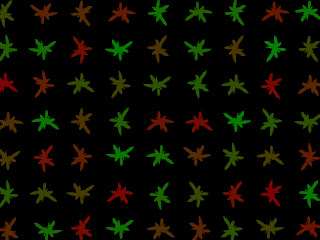Figure 1. Seedling silhouettes are colored various shades of red, green, and brown because I think the diagrams are more interesting if they have multiple colors in them. Also, one parent is red, and one parent is green, so at least in theory, the offspring could wind up anywhere in between the two, which makes it almost realistic or something.

Question 2. How many rows and columns of seeds should he make if he wants to place the seeds as far apart from one another as possible, but in a triangular/hexagonal grid instead, as in Figure 2?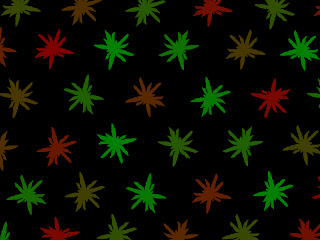Figure 2

Extra credit. How many of the 38 seeds Mr. Subjunctive plants will actually germinate and grow long enough to flower and produce their own seeds? Explain your answer.

Answers are below.

ANSWERS:

Question 1. Although we could just plant a single row of 38 seedlings down the center of the rectangle and call it done, the seedlings would have to be very close together in the row, with a lot of wasted space on either side, so they'll crowd one another out. In order to give each seed as much space as possible, we're going to use algebra to try and find a rectangle of seedlings that has the proportions which best fit the container.

Since we know that the container is a rectangle, 12 inches by 8 inches, we know that we're looking for a rectangle of seedlings with proportions similar to 12 x 8.

So. Let x = the number of seedlings in a column, parallel to the short side of the container. The number of seedlings in a row (parallel to the long side of the container) then has to be 1.5x, because the long side of the container is (12/8) = 1.5 times longer than the short side.

To solve for x and find out how many seedlings to plant per column, we multiply x (the number per column) with 1.5x (the number per row) and set that equal to 38 (the total number of seedlings we have):

38 = 1.5x2

Dividing both sides by 1.5:

25.3 = x2

Taking the square root of both sides to solve for x:

x = √(25.3) = 5 (more or less)

(3/2)x = 7.5 (more or less)

So the most evenly-spaced gridded arrangement would be five rows of eight seedlings, with two empty spaces somewhere in the grid, as in Figure 3:Figure 3. Solution to Question 1.

Question 2. Although the whole triangular-grid thing is unusual and scary, we can look at this as just the sum of two rectangles. See Figure 4.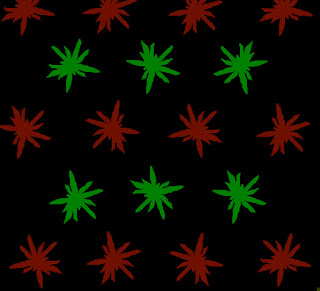Figure 4. Here, the red seedlings are arranged as a 4x3 rectangle, while the green seedlings are in a 3x2 rectangle. When looking at the red and green seedlings combined, though, they're still in a triangular grid: we're just looking at them as two separate rectangles, added together, because that makes it possible to calculate what we need to calculate.

The outer rectangle will have x rows and y columns; the inner rectangle will then have (x-1) rows and (y-1) columns because . . . it just kind of has to?2

So, the seedlings in the outer rectangle = xy.

The seedlings in the inner rectangle = (x-1)(y-1) = xy-x-y+1.

The sum of the seedlings in the two rectangles combined should be equal to the number of seedlings we have, so:

38 = (xy) + (xy-x-y+1)
38 = xy+xy-x-y+1
38 = 2xy-x-y+1

Well. We're not going to be able to solve it looking like that. So let's think about what we know of x and y, compared to one another.

If we call the short side y, then we know that x=1.5y, using the same reasoning as in the first question. So, everywhere that x appears in the original sum, we can substitute 1.5y instead, giving us this:

38 = 2(1.5y)y-(1.5y)-y+1
38 = 3y2-1.5y-y+1
38 = 3y2-2.5y+1

Subtracting 38 from both sides:

0 = 3y2-2.5y-37

Which is a quadratic equation, and those are easily solved in the internet age.3 Putting in the numbers a=3, b=-2.5, and c=-37, the formula gives two possible answers for y:

3.953 blah blah blah, and
-3.120 blah blah blah.

As interesting as it might be to try to plant negative three rows of seedlings, or 3.95 rows, for that matter, for our purposes there's only one valid answer, which is that y is basically equal to 4.

If y=4, then x has to be (1.5)(4) = 6.

So the large rectangle is 6 columns by 4 rows --Figure 6

Making the small rectangle 5 columns by 3 rows --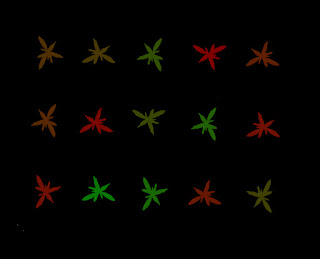Figure 7

Which means 24 in the large rectangle and 15 in the small rectangle. 24 plus 15 is 39, and we only have 38 seedlings, so one of the holes will have to be empty, as in figure 8.Figure 8. Solution to Question 2.

Extra credit.
The answer is zero, because Mr. Subjunctive has never attempted to germinate Cryptbergia seeds before, and couldn't find anything on the internet to tell him how to do it, so he's probably going about this completely wrong. There's a possibility that the seeds were already dead by the time he noticed them, also.

I would also accept: zero, because however many of them germinate and grow, they're just going to get scale, thrips, or fungus and be thrown out anyway.
Special note regarding algebra: all kidding aside, though, algebra is way more useful in day to day life than you might think. Trigonometry is way overrated, and I don't think I've ever needed calculus or differential equations for anything outside of schoolwork (which is lucky, 'cause I no longer remember how to do any of it), and arithmetic is trivial, what with calculators and Google and all,4 but algebra comes up all the time and really is just as useful as your algebra teachers always told you it would be.
-

1 What kind of seeds? Well, if you must know, I tried to cross the Cryptbergia x rubra with the Billbergia nutans that was blooming at the same time, making them technically some sort of Cryptbergia. Let's call them Cryptbergia x subjunctiva. Here's what the dried inflorescences looked like:And here is what the seeds looked like, with the dead flower parts still attached: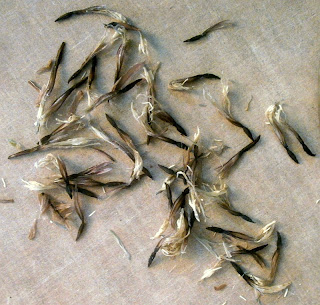The kind of seeds doesn't actually matter to the math, though, obviously.
2 I know there's nothing in the whole triangular-grid concept that would prevent us from extending the inner rectangle on one side, like in Figure 5:Figure 5. Here we've got the same 4x3 rectangle of red seedlings, but now there's a 3x3 rectangle of green ones, instead of 3x2, because the green rectangle is not entirely enclosed by the red one. Instead, one row of green ones is outside the rectangle of red ones.

Which since you're so smart, why don't you just go ahead and figure out how to arrange the seedlings if the inner rectangle is extended on one side then. That oughta teach you a lesson about thinking independently.
3 This is the point where most math teachers would include something about the quadratic equation. While it's true that the quadratic equation can be a handy thing to know sometimes, 1) because of the internet, it's never more than a Google search away, so memorizing it is a lot less valuable than it used to be, and 2) Mr. Subjunctive isn't asking you this question because he cares about whether or not you know the quadratic equation; he just cares about how to arrange the holes he's going to poke in the vermiculite. No need to churn your butter by hand when you can just go to the supermarket.
4 In case you didn't know: you can type arithmetic problems ("2*8+13") directly into Google searches now, and it'll give you the answer ("29"). This is frequently more trouble than just using my trusty TI-81 calculator, so I don't actually Google for arithmetic that often, but still. Nice to know the option is there.

#### 5 comments:

Ed Kramer said...

I got mixed bromeliad seeds from Park or T&M many years ago and the instructions said to surface sow.

mr_subjunctive said...

Ed Kramer:

That's basically what I did; I only made shallow holes in the vermiculite so I could see where I was supposed to put them. None of them are covered.

Did the seeds you got look at all like these? Is there any reason for me to think that they might be viable?

Ed Kramer said...

I think they did look like that. If they developed any thickness i think they might be good.

Liza said...

How was that a word problem? All those numbers make me dizzy! Juuust kidding. I hope your seeds make it!Anonymous said...

I once wet myself in terror when confronted with a problem like that in grade 3. In front of the whole class. I'd managed to suppress the incident until now. Thanks a bunch Mr.S! You're swell.

Jenny